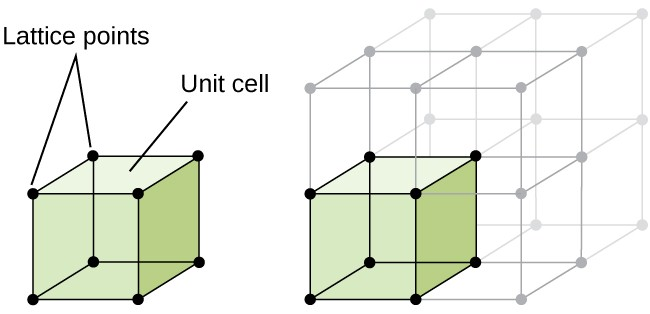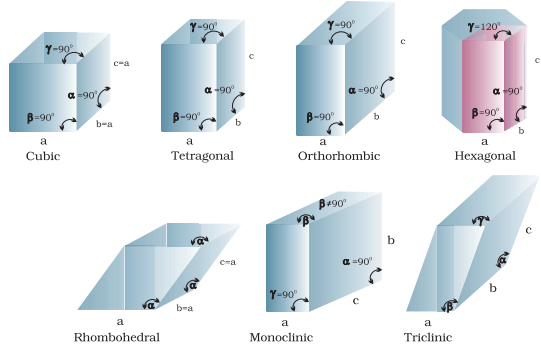×#### Thank you for registering.

One of our academic counsellors will contact you within 1 working day.

Click to Chat

1800-1023-196

+91-120-4616500

CART 0

• 0

MY CART (5)

Use Coupon: CART20 and get 20% off on all online Study Material

ITEM
DETAILS
MRP
DISCOUNT
FINAL PRICE
Total Price: Rs.

There are no items in this cart.
Continue Shopping```Crystal Lattices and Unit Cells

Crystal Lattice

Characteristics of Crystal Lattices

Unit Cell

Primitive Unit Cells

Centred Unit Cells

Formation of Lattice

Crystal Lattice

Crystal Lattice is a three-dimensional representation of atoms and molecules arranged in a specific order/pattern. In other words, a crystal lattice can be defined as a geometrical arrangement of constituent particles of matter (atoms, ions or molecules) as points in space. There are total 14 possible three-dimensional lattices. Crystal lattices are also known by Bravais Lattices, named after the scientist Auguste Bravais.Image 1: Example of a crystal lattice

Characteristics of Crystal Lattices

The following characteristics are depicted by Bravais lattices:

Each point in a lattice represents a lattice site or we can say lattice point

Each point denotes a particular type of constituent particles of matter be it an atom, molecule or an ion

By joining the lattices points inside the lattice we can define geometry of the lattice

Unit CellImage 2: A unit cell is the shortest portion of a lattice

The smallest possible portion or part of the crystal lattice which repeats itself in different directions of the lattice is called the unit cell. Many unit cells combine to geometrically form the crystal lattice.

Characteristics of Unit Cell

The following characteristics define a unit cell:

A unit cell has three edges a, b and c and three angles α, β and γ between the respective edges

The a, b and c may or may not be mutually perpendicular

The angle between edge b and c is α, a and c is β and that of between a and b is γ

Unit cells are of two types namely:

Primitive Unit Cells

Centred Unit CellsImage 3: Types of Unit Cells

Primitive Unit Cells

The unit cell in which the constituent particles (atoms, ions or molecules) are located only on the corners of the lattice is called A Primitive Unit Cell.

Centred Unit CellsImage 4: End Centred Cell

The unit cell in which the constituent particles (atoms, ions or molecules) are located on the corners, as well as other positions of the lattice, is known as Centred Unit Cells. A centred unit cell is further divided into three types:

Body Centred Unit Cells

Face Centred Unit Cells

End Centred Unit Cells

Body Centred Unit Cells

The unit cell which contains one constituent particle (atom, molecule or ion) at its body centre and other constituent particles are located on the corners is called Body Centred Unit Cells.

Face Centred Unit Cells

The unit cell which contains constituent particles (atoms, molecules or ions) on each face of the unit cell and other constituent particles on the corners is called the Face Centred Unit Cell.

End Centred Unit Cells

In an end centred unit cell, one constituent particle (atom, molecule or ion) is present at the centre of opposite faces besides the ones located on the corners.

Formation of LatticeImage 5: Types of unit cells which form crystal lattices

Formation of unit cells takes place in seven forms, namely:

Cubic Lattice

Tetragonal Lattice

Orthorhombic Lattice

Monoclinic Lattice

Hexagonal Lattice

Rhombohedral Lattice

Triclinic Lattice

Cubic Lattice: Cubic lattice is formed into three possible geometries of unit cells: primitive, body-centred and face centred unit cells. In a cubic lattice, all the edges are equal and the angle between their faces is 90° that is, mutually perpendicular.

Tetragonal Lattice: The formation of tetragonal lattice takes place in two geometries of unit cells: primitive and body centred unit cells. In a tetragonal lattice, only one edge has different length and angle between respective edges is 90° that is, mutually perpendicular

Orthorhombic Lattice: There are four types of orthorhombic lattice mainly: primitive, end-centred, body-centred and face centred. In orthorhombic lattice, the edge lengths are unequal in nature and the angle between respective edges is 90° that is, mutually perpendicular

Monoclinic Lattice: Monoclinic lattice is formed from two types of unit cells namely: primitive and end centred. Monoclinic lattice has unequal sides and two angles between the faces of the lattice are 90°.

Hexagonal Lattice: Hexagonal lattice is formed from only one type of unit cell that is, primitive. In hexagonal lattice, only one side and two angles are 90° and one angle is 120°.

Rhombohedral Lattice: Rhombohedral Lattice is also formed from one type of unit cell that is,  primitive. In Rhombohedral Lattice all the sides are equal and two angles between the faces of the rhombohedral lattice are less than 90°.

Triclinic Lattice: The formation of triclinic lattice also takes place from one type of unit cell that is, primitive. In triclinic lattice all the sides are unequal and none of the angles between the faces of the triclinic lattice are 90°.

The table given below can be used to summarize types of lattice formation.

Lattice

Types

Edge Length

Angles between faces

Examples

Cubic

Primitive, Body-centred, Face-centred

a = b = c

α =  β = γ = 90°

NaCl, Copper and ZnS

Tetragonal

Primitive, Body-centred

a = b ≠ c

α =  β = γ = 90°

White tin, SnO2, TiO2 and CaSO4

Orthorhombic

Primitive, Body-centred, Face-centred , End-centred

a ≠ b ≠ c

α =  β = γ = 90°

Rhombic Sulphur, BaSO4 and KNO3

Hexagonal

Primitive

a = b ≠ c

α =  β = 90° and γ = 120°

Graphite, ZnO and CdS

Rhombohedral

Primitive

a = b = c

α =  β = γ ≠ 90°

CaCO3 ( Calcite) and HgS (cinnabar)

Monoclinic

Primitive, End-centred

a ≠ b ≠ c

α = γ = 90° β ≠ 90°

Sulphur

Triclinic

Primitive

a ≠ b ≠ c

α ≠  β ≠ γ ≠ 900

H3PO3 , CuSO4.5 H2O

Watch this Video for more reference

Crystal Lattices and Unit Cells
```### Course Features

• 731 Video Lectures
• Revision Notes
• Previous Year Papers
• Mind Map
• Study Planner
• NCERT Solutions
• Discussion Forum
• Test paper with Video Solution